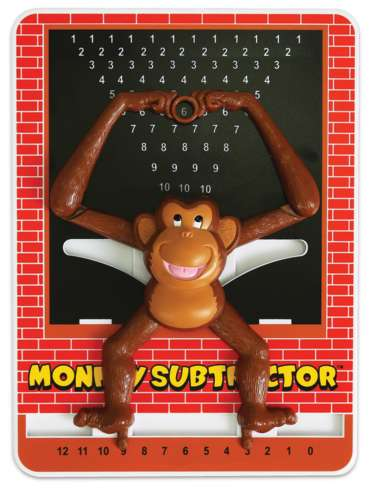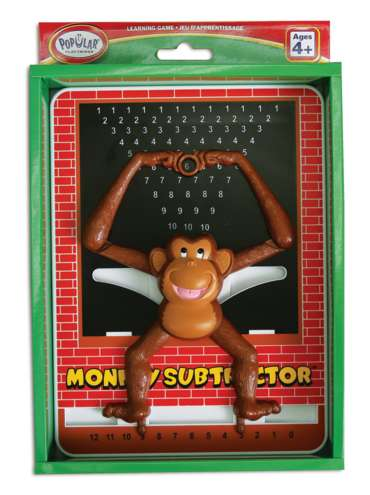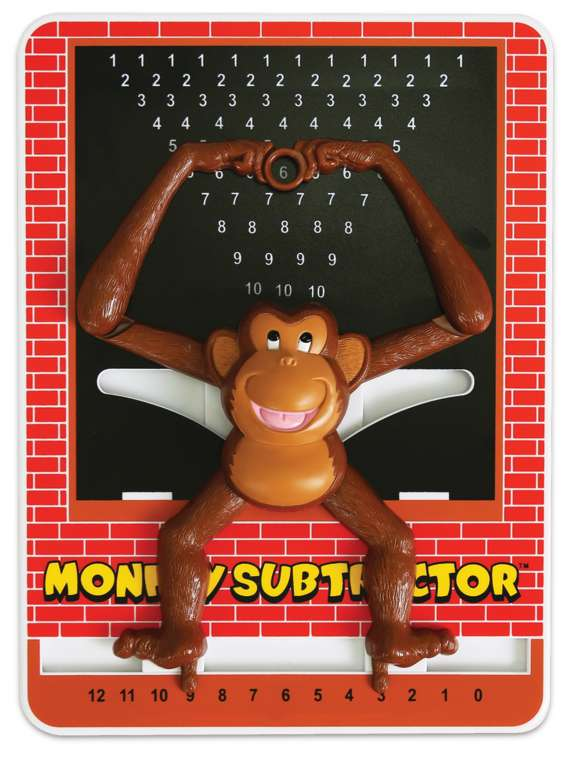••# Monkey Subtractor™ Calculator

Product Number: PPY50203
\$9.99
Children will have fun learning simple arithmetic with these large 8.5 x 11" monkey calculators. Choose the Monkey Subtractor...
Quantity

Children will have fun learning simple arithmetic with these large 8.5 x 11" monkey calculators. Choose the Monkey Subtractor for help with subtraction. Then slide the monkey's feet so his toes point to the numbers you want to subtract and the correct answer will appear in the ring between the monkey's hands!